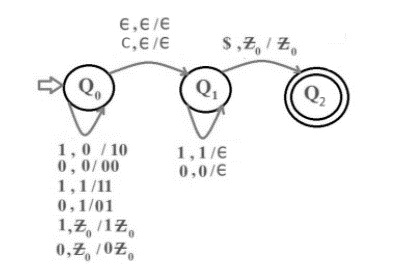# Construct Pushdown Automata for all length palindromes

L = {ww’ | wcw’, w={0, 1}*} where w’ is the reverse of w.

This is the language of all palindromes, both odd and even over the alphabet {0,1}.

For the construction of all length palindromes, let us use the Non-deterministic push down automata (NPDA).

To construct the wcw’ we need to check if the string is of odd length and if reaches the middle element ‘c’ then process it and move to the next state without making any change in stack.

## Example

Given string is 1 1 0 0 1 1 1 1 0 0 1 1

Result − ACCEPTED

Given string is: 1 0 1 0 1 0 1 0 1

Result − ACCEPTED

The transition diagram is as follows−Step 1 − On receiving 0 or 1, push the elements on top of stack and check if input string is of even length then whether it reach to second half of input string or not,

Suppose, if the input string is of odd length then check whether it reaches the middle element or not.

Step 2 − If input string is of even length and reach to last element of first half of input string, then push that element on top of stack and make an epsilon move to next state or Suppose, if the input string is of odd length then on receiving an element ‘c’, move to the next state without making any change in stack.

Step 3 − On receiving an element, check if symbol scanned if symbol scanned is ‘0’ and top of stack also contain ‘0’ or ‘1’ and top of stack also contain ‘1’ then pop the element from top of stack else move to dead state.

Repeat step 3 until the string becomes empty.

Step 4 − Check if the symbol scanned is ‘\$’ and the stack does not contain any element then move to final state or else move to dead state.